# NCERT Solutions for Class 9th: Ch 8 Motion Science

#### NCERT Solutions for Class 9th: Ch 8 Motion Science

In Text Question

Page No: 100

1. An object has moved through a distance. Can it have zero displacement? If yes, support your answer with an example.

Yes,an object can have zero displacement even when it has moved through a distance.This happens when final position of the object coincides with its initial position. For example,if a person moves around park and stands on place from where he started then here displacement will be zero.

2. A farmer moves along the boundary of a square field of side 10 m in 40 s. What will be the magnitude of displacement of the farmer at the end of 2 minutes 20 seconds from his initial position?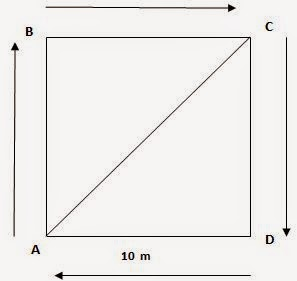Given, Side of the square field= 10m
Therefore, perimeter = 10 m × 4 = 40 m
Farmer moves along the boundary in 40s.
Displacement after 2 m 20 s = 2 × 60 s + 20 s = 140 s =?
Since in 40 s farmer moves 40 m
Therefore, in 1s distance covered by farmer = 40 / 40 m = 1m
Therefore, in 140s distance covered by farmer = 1 × 140 m = 140 m. Now, number of rotation to cover 140 along the boundary= Total Distance / Perimeter
= 140 m /40 m  = 3.5 round
Thus, after 3.5 round farmer will at point C of the field.

Thus, after 2 min 20 seconds the displacement of farmer will be equal to 14.14 m north east from intial position.

3. Which of the following is true for displacement?
(a) It cannot be zero.
(b) Its magnitude is greater than the distance travelled by the object.

None of the statement is true for displacement First statement is false because displacement can be zero. Second statement is also false because displacement is less than or equal to the distance travelled by the object.

Page No: 102

1. Distinguish between speed and velocity.

 Speed Velocity Speed is the distance travelled by an object in a given interval of time. Velocity is the displacement of an object in a given interval of time. Speed = distance / time Velocity = displacement / time Speed is scalar quantity i.e. it has only magnitude. Velocity is vector quantity i.e. it has both magnitude as well as direction.

2. Under what condition(s) is the magnitude of average velocity of an object equal to its average speed?

The magnitude of average velocity of an object is equal to its average speed, only when an object is moving in a straight line.

3. What does the odometer of an automobile measure?

The odometer of an automobile measures the distance covered by an automobile.

4. What does the path of an object look like when it is in uniform motion?

An object having uniform motion has a straight line path.

5. During an experiment, a signal from a spaceship reached the ground station in five minutes. What was the distance of the spaceship from the ground station? The signal travels at the speed of light, that is, 3 × 108 m s−1.

Speed= 3 × 108 m s−1
Time= 5 min = 5 × 60 = 300 secs.
Distance= Speed × Time
Distance= 3 × 108 m s−1 × 300 secs. = 9 × 1010 m

Page No: 103

1. When will you say a body is in (i) uniform acceleration? (ii) non-uniform acceleration?

(i) A body is said to be in uniform acceleration if it travels in a straight line and its velocity increases or decreases by equal amounts in equal intervals of time.
(ii) A body is said to be in nonuniform acceleration if the rate of change of its velocity is not constant.

2. A bus decreases its speed from 80 km h−1 to 60 km h−1 in 5 s. Find the acceleration of the bus.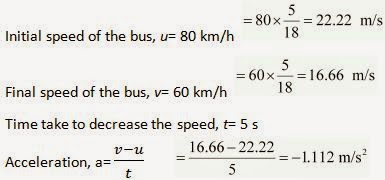3. A train starting from a railway station and moving with uniform acceleration attains a speed 40 km h−1 in 10 minutes. Find its acceleration.

Page No: 107

1. What is the nature of the distance - 'time graphs for uniform and non-uniform motion of an object?

When the motion is uniform,the distance time graph is a straight line with a slope.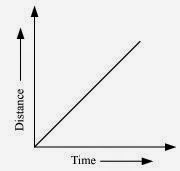When the motion is non uniform, the distance time graph is not a straight line.It can be any curve.2. What can you say about the motion of an object whose distance - time graph is a straight line parallel to the time axis?

If distance time graph is a straight line parallel to the time axis, the body is at rest.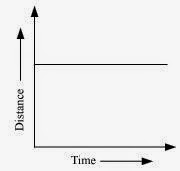3. What can you say about the motion of an object if its speed - 'time graph is a straight line parallel to the time axis?

If speed time graph is a straight line parallel to the time axis, the object is moving uniformly.4. What is the quantity which is measured by the area occupied below the velocity -time graph?

The area below velocity-time graph gives the distance covered by the object.

Page No: 109

1. A bus starting from rest moves with a uniform acceleration of 0.1 m s−2 for 2 minutes. Find (a) the speed acquired, (b) the distance travelled.

Initial speed of the bus, u= 0
Acceleration, a = 0.1 m/s2
Time taken, t = 2 minutes = 120 s

(a) v= u + at
v= 0 + 0.1 × 120
v= 12 ms–1

(b) According to the third equation of motion:
v2 - u2= 2as
Where, s is the distance covered by the bus
(12)2 - (0)2= 2(0.1) s
s = 720 m

Speed acquired by the bus is 12 m/s.
Distance travelled by the bus is 720 m.

Page No: 110

2. A train is travelling at a speed of 90 km h−1. Brakes are applied so as to produce a uniform acceleration of −0.5 m s−2. Find how far the train will go before it is brought to rest.

Initial speed of the train, u= 90 km/h = 25 m/s
Final speed of the train, v = 0 (finally the train comes to rest)
Acceleration = - 0.5 m s-2
According to third equation of motion:
v2= u2+ 2 as
(0)2= (25)2+ 2 ( - 0.5) s

Where, s is the distance covered by the train

The train will cover a distance of 625 m before it comes to rest.

3. A trolley, while going down an inclined plane, has an acceleration of 2 cm s−2. What will be its velocity 3 s after the start?

Initial Velocity of trolley, u= 0 cms-1
Acceleration, a= 2 cm s-2
Time, t= 3 s
We know that final velocity, v= u + at = 0 + 2 x 3 cms-1
Therefore, The velocity of train after 3 seconds = 6 cms-1

4. A racing car has a uniform acceleration of 4 m s - '2. What distance will it cover in 10 s after start?

Initial Velocity of the car, u=0 ms-1
Acceleration, a= 4 m s-2
Time, t= 10 s
We know Distance, s= ut + (1/2)at2
Therefore, Distance covered by car in 10 second= 0 × 10 + (1/2) × 4 × 102
= 0 + (1/2) × 4 × 10 × 10 m
= (1/2) × 400 m
= 200 m

5. A stone is thrown in a vertically upward direction with a velocity of 5 m s−1. If the acceleration of the stone during its motion is 10 m s−2 in the downward direction, what will be the height attained by the stone and how much time will it take to reach there?

Given Initial velocity of stone, u=5 m s-1
Downward of negative Acceleration, a= 10 m s-2
We know that 2 as= v2- u2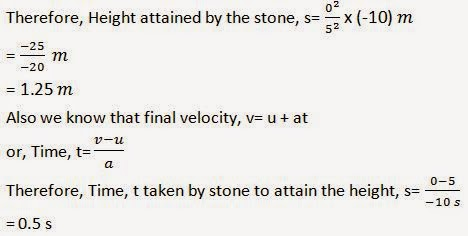Page No: 112

Excercise

1. An athlete completes one round of a circular track of diameter 200 m in 40 s. What will be the distance covered and the displacement at the end of 2 minutes 20 s?

Diameter of circular track (D) = 200 m
Radius of circular track (r) = 200 / 2=100 m
Time taken by the athlete for one round (t) = 40 s
Distance covered by athlete in one round (s) = 2π r
= 2 × ( 22 / 7 ) × 100
Speed of the athlete (v) = Distance / Time
= (2 × 2200) / (7 × 40)
= 4400 / 7 × 40
Therefore, Distance covered in 140 s = Speed (s) × Time(t)
= 4400 / (7 × 40) × (2 × 60 + 20)
= 4400 / (7 × 40) × 140
= 4400 × 140 /7 × 40
= 2200 m

Number of round in 40 s =1 round
Number of round in 140 s =140/40
=3 1/2
After taking start from position X,the athlete will be at postion Y after 3 1/2 rounds as shown in figureHence, Displacement of the athlete with respect to initial position at x= xy
= Diameter of circular track
= 200 m

2.  Joseph jogs from one end A to the other end B of a straight 300 m road in 2 minutes 30 seconds and then turns around and jogs 100 m back to point C in another 1 minute. What are Joseph's average speeds and velocities in jogging (a) from A to B and (b) from A to C?

Total Distance covered from AB = 300 m
Total time taken = 2 × 60 + 30 s
=150 sTherefore, Average Speed from AB = Total Distance / Total Time
=300 / 150 m s-1
=2 m s-1
Therefore, Velocity from AB =Displacement AB / Time = 300 / 150 m s-1
=2 m s-1
Total Distance covered from AC =AB + BC
=300 + 200 m

Total time taken from A to C = Time taken for AB + Time taken for BC
= (2 × 60+30)+60 s
= 210 s
Therefore, Average Speed from AC = Total Distance /Total Time
= 400 /210 m s-1
= 1.904 m s-1

Displacement (S) from A to C = AB - BC
= 300-100 m
= 200 m

Time (t) taken for displacement from AC = 210 s

Therefore, Velocity from AC = Displacement (s) / Time(t)
= 200 / 210 m s-1
= 0.952 m s-1

3. Abdul, while driving to school, computes the average speed for his trip to be 20 km h−1. On his return trip along the same route, there is less traffic and the average speed is 40 km h−1. What is the average speed for Abdul’s trip?

The distance Abdul commutes while driving from Home to School = S
Let us assume time taken by Abdul to commutes this distance = t1
Distance Abdul commutes while driving from School to Home = S
Let us assume time taken by Abdul to commutes this distance = t2
Average speed from home to school v1av = 20 km h-1
Average speed from school to home v2av = 30 km h-1
Also we know Time taken form Home to School t1 =S / v1av
Similarly Time taken form School to Home t2 =S/v2av
Total distance from home to school and backward = 2 S
Total time taken from home to school and backward (T) = S/20+ S/30
Therefore, Average speed (Vav) for covering total distance (2S) = Total Distance/Total Time
= 2S / (S/20 +S/30)
= 2S / [(30S+20S)/600]
= 1200S / 50S
= 24 kmh-1

4. A motorboat starting from rest on a lake accelerates in a straight line at a constant rate of 3.0 m s−2 for 8.0 s. How far does the boat travel during this time?

Given Initial velocity of motorboat,  u = 0
Acceleration of motorboat,  a = 3.0 m s-2
Time under consideration,  t = 8.0 s
We know that Distance, s = ut + (1/2)at2
Therefore, The distance travel by motorboat = 0 ×8 + (1/2)3.0 × 82
= (1/2) × 3 × 8 × 8 m
= 96 m

5. A driver of a car travelling at 52 km h−1 applies the brakes and accelerates uniformly in the opposite direction. The car stops in 5 s. Another driver going at 3 km h−1 in another car applies his brakes slowly and stops in 10 s. On the same graph paper, plot the speed versus time graphs for the two cars. Which of the two cars travelled farther after the brakes were applied?

As given in the figure below PR and SQ are the Speed-time graph for given two cars with initial speeds 52 kmh−1 and 3 kmh−1 respectively.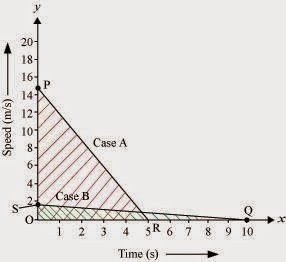Distance Travelled by first car before coming to rest =Area of △ OPR
= (1/2) × OR × OP
= (1/2) × 5s × 52 kmh−1
= (1/2) × 5 × (52 × 1000) / 3600) m
= (1/2) × 5 × (130 / 9) m
= 325 / 9 m
= 36.11 m
Distance Travelled by second car before coming to rest =Area of △ OSQ
= (1/2) × OQ × OS
= (1/2) × 10 s × 3 kmh−1
= (1/2) × 10 × (3 × 1000) / 3600) m
= (1/2) × 10 x (5/6) m
= 5 × (5/6) m
= 25/6 m
= 4.16 m

6. Fig 8.11 shows the distance-time graph of three objects A, B and C. Study the graph and answer the following questions: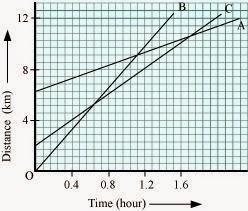(a) Which of the three is travelling the fastest?
(b) Are all three ever at the same point on the road?
(c) How far has C travelled when B passes A?
(d)How far has B travelled by the time it passes C?

(a) Object B
(b) No
(c) 5.714 km
(d) 5.143 kmTherefore, Speed = slope of the graph
Since slope of object B is greater than objects A and C, it is travelling the fastest.

(b) All three objects A, B and C never meet at a single point. Thus, they were never at the same point on road.

(c)7 square box = 4 km
∴ 1 square box = 4/7 km
C is 4 blocks away from origin therefore initial distance of C from origin = 16/7 km
Distance of C from origin when B passes A = 8 km
Thus, Distance travelled by C when B passes A = 8 - 16/7 = (56 - 16)/7 = 40/7 = 5.714 km

(d)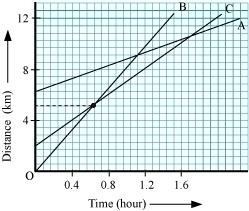Distance travelled by B by the time it passes C = 9 square boxes
9×4/7 = 36/7 = 5.143 km

Page No: 113

7. A ball is gently dropped from a height of 20 m. If its velocity increases uniformly at the rate of 10 m s−2, with what velocity will it strike the ground? After what time will it strike the ground?

Let us assume, the final velocity with which ball will strike the ground be 'v' and time it takes to strike the ground be 't'
Initial Velocity of ball,  u =0
Distance or height of fall,  s =20 m
Downward acceleration,  a =10 m s-2
As we know, 2as =v2-u2
v2 = 2as+ u2
= 2 x 10 x 20 + 0
= 400
∴ Final velocity of ball, v = 20 ms-1
t = (v-u)/a
∴Time taken by the ball to strike = (20-0)/10
= 20/10
= 2 seconds

8. The speed-time graph for a car is shown is Fig. 8.12.(a) Find out how far the car travels in the first 4 seconds. Shade the area on the graph that represents the distance travelled by the car during the period.
(b) Which part of the graph represents uniform motion of the car?

(a)The shaded area which is equal to 1/2 × 4 × 6 = 12 m represents the distance travelled by the car in the first 4 s.

(b)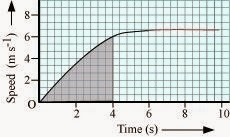The part of the graph in red colour between time 6 s to 10 s represents uniform motion of the car.

9. State which of the following situations are possible and give an example for each of these:
(a) an object with a constant acceleration but with zero velocity.
(b) an object moving in a certain direction with an acceleration in the perpendicular direction.

(a) Possible
When a ball is thrown up at maximum height, it has zero velocity, although it will have constant acceleration due to gravity, which is equal to 9.8 m/s2.

(b) Possible
When a car is moving in a circular track, its acceleration is perpendicular to its direction.

10. An artificial satellite is moving in a circular orbit of radius 42250 km. Calculate its speed if it takes 24 hours to revolve around the earth.